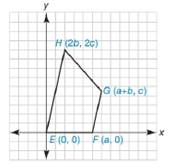Chapter 10.2, Problem 41E### Elementary Geometry for College St...

6th Edition
Daniel C. Alexander + 1 other
ISBN: 9781285195698

#### Solutions

Chapter
Section### Elementary Geometry for College St...

6th Edition
Daniel C. Alexander + 1 other
ISBN: 9781285195698
Textbook Problem
70 views

# Quadrilateral E F G H has the vertices E 0 ,   0 , F a ,   0 , G a + b ,   c , and H 2 b , 2 c . Verify that E F G H is a trapezoid by showing that the slopes of two sides are equal.To determine

To prove:

The quadrilateral EFGH is trapezoid by showing the slopes of two sides are equal.Explanation

To prove that the quadrilateral is a trapezoid, one of the opposite sides must be parallel,

By theorem,

If two lines are parallel, then their slopes are equal.

(i.e) If l1l2, then m1=m2.

So we can prove this by using the slopes.

Thus we have to prove the line EH is parallel to the line FG.

The slope of the line that contains the points x1,y1 and x2,y2 is given by

m=y2-y1x2-x1 for x2x1

Let mEH- and mFG- are the slopes of the line EH- and FG- respectively.

The given vertices are E0, 0, and H2b, 2c.

Using the slope formula and choosing x1=0, x2=2b, y1=0, and y2=2c

### Still sussing out bartleby?

Check out a sample textbook solution.

See a sample solution

#### The Solution to Your Study Problems

Bartleby provides explanations to thousands of textbook problems written by our experts, many with advanced degrees!

Get Started

#### Find more solutions based on key concepts